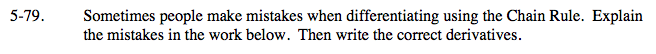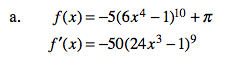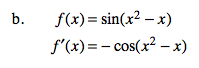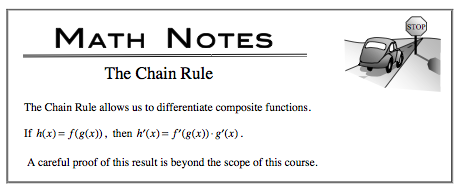### Home > CALC > Chapter 5 > Lesson 5.2.3 > Problem5-79

5-79.
1. Sometimes people make mistakes when differentiating using the Chain Rule. Explain the mistakes in the work below. Then write the correct derivatives. Homework Help ✎

1. f(x) = −5(6x4 − 1)10 + π
f ′(x) = −50(24x3 − 1)9

2. f(x) = sin(x2x)
f ′(x) = − cos(x2x)